# Homomorphic filtering – part 1

I'd like to welcome back guest blogger Spandan Tiwari for today's post. Spandan, a developer on the Image Processing Toolbox team, posted here previously about circle finding.

Recently I attended a friend's wedding at an interesting wedding venue, the Charles River Museum of Industry and Innovation in the town of Waltham, not very far from the Mathworks headquarters here in Natick. While waiting for the guests to arrive, we all got a chance to look around the museum. It's a nice little place with several quirky old machines on display, some dating back to 1812. Hanging on a wall in a dimly lit corner of the museum was a framed copy of an engineer's decadic logarithm (and anti-logarithm) tables. A friend who was looking around with me commented, "Ah, logs...haven't seen these since school days." That got me thinking. When was the last time I used logarithms? Well, just in the morning I had used the log function for scaling the Fourier magnitude spectrum of an image for better visualization. It took me a moment though to make the mental connection between the printed log tables and log's functional form, but as soon as I did, I realized that I used log quite often.

It also reminded me of a neat image enhancement technique from the olden days of image processing called homomorphic filtering, which makes clever use of the log function. There are several image denoising/enhancement techniques in literature that assume an additive noise model, i.e. $\tilde I(x,y) = I(x,y) + n(x,y)$, where $n$ is the noise signal. But there are relatively few techniques that work with other noise models, such as the multiplicative model $\tilde I(x,y) = I(x,y)\ n(x,y)$.

Homomorphic filtering is one such technique for removing multiplicative noise that has certain characteristics.

Homomorphic filtering is most commonly used for correcting non-uniform illumination in images. The illumination-reflectance model of image formation says that the intensity at any pixel, which is the amount of light reflected by a point on the object, is the product of the illumination of the scene and the reflectance of the object(s) in the scene, i.e.,

$$I(x,y) = L(x,y)\ R(x,y)$$

where $I$ is the image, $L$ is scene illumination, and $R$ is the scene reflectance. Reflectance $R$ arises from the properties of the scene objects themselves, but illumination $L$ results from the lighting conditions at the time of image capture. To compensate for the non-uniform illumination, the key is to remove the illumination component $L$ and keep only the reflectance component $R$. If we consider illumination as the noise signal (which we want to remove), this model is similar to the multiplicative noise model shown earlier.

Illumination typically varies slowly across the image as compared to reflectance which can change quite abruptly at object edges. This difference is the key to separating out the illumination component from the reflectance component. In homomorphic filtering we first transform the multiplicative components to additive components by moving to the log domain.

$$\ln(I(x,y)) = \ln(L(x,y)\ R(x,y))$$

$$\ln(I(x,y)) = \ln(L(x,y)) + \ln(R(x,y))$$

Then we use a high-pass filter in the log domain to remove the low-frequency illumination component while preserving the high-frequency reflectance component. The basic steps in homomorphic filtering are shown in the diagram below: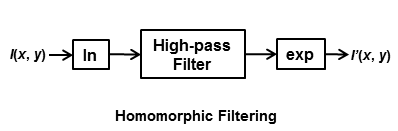For a working example I will use an image from the Image Processing Toolbox.

I = imread('AT3_1m4_01.tif');
imshow(I)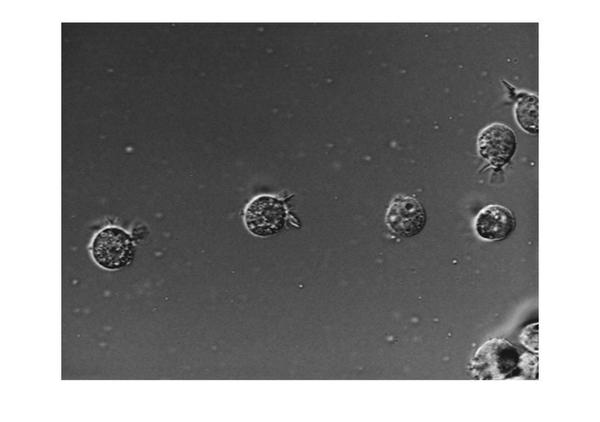In this image the background illumination changes gradually from the top-left corner to the bottom-right corner of the image. Let's use homomorphic filtering to correct this non-uniform illumination.

The first step is to convert the input image to the log domain. Before that we will also convert the image to floating-point type.

I = im2double(I);
I = log(1 + I);


The next step is to do high-pass filtering. We can do high-pass filtering in either the spatial or the spectral domain. Although they are both exactly equivalent, each domain offers some practical advantages of its own. We will do both it ways. Let's start with frequency-domain filtering. In frequency domain the homomorphic filtering process looks like: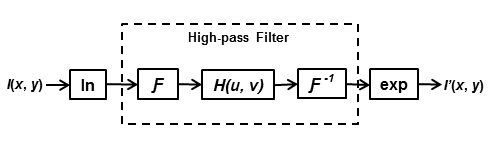First we will construct a frequency-domain high-pass filter. There are different types of high-pass filters you can construct, such as Gaussian, Butterworth, and Chebychev filters. We will construct a simple Gaussian high-pass filter directly in the frequency domain. In frequency domain filtering we have to careful about the wraparound error which comes from the fact that Discrete Fourier Transform treats a finite-length signal (such as the image) as an infinite-length periodic signal where the original finite-length signal represents one period of the signal. Therefore, there is interference from the non-zero parts of the adjacent copies of the signal. To avoid this, we will pad the image with zeros. Consequently, the size of the filter will also increase to match the size of the image.

M = 2*size(I,1) + 1;
N = 2*size(I,2) + 1;


Note that we can make the size of the filter (M,N) even numbers to speed-up the FFT computation, but we will skip that step for the sake of simplicity. Next, we choose a standard deviation for the Gaussian which determines the bandwidth of low-frequency band that will be filtered out.

sigma = 10;


And create the high-pass filter...

[X, Y] = meshgrid(1:N,1:M);
centerX = ceil(N/2);
centerY = ceil(M/2);
gaussianNumerator = (X - centerX).^2 + (Y - centerY).^2;
H = exp(-gaussianNumerator./(2*sigma.^2));
H = 1 - H;


Couple of things to note here. First, we formulate a low-pass filter and then subtracted it from 1 to get the high-pass filter. Second, this is a centered filter in that the zero-frequency is at the center.

imshow(H,'InitialMagnification',25)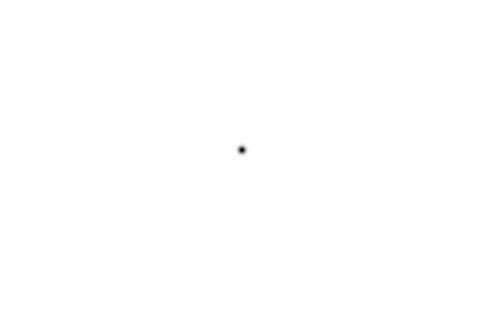We can rearrange the filter in the uncentered format using fftshift.

H = fftshift(H);


Next, we high-pass filter the log-transformed image in the frequency domain. First we compute the FFT of the log-transformed image with zero-padding using the fft2 syntax that allows us to simply pass in size of the padded image. Then we apply the high-pass filter and compute the inverse-FFT. Finally, we crop the image back to the original unpadded size.

If = fft2(I, M, N);
Iout = real(ifft2(H.*If));
Iout = Iout(1:size(I,1),1:size(I,2));


The last step is to apply the exponential function to invert the log-transform and get the homomorphic filtered image.

Ihmf = exp(Iout) - 1;


Let's look at the original and the homomorphic-filtered images together. The original image is on the left and the filtered image is on the right. If you compare the two images you can see that the gradual change in illumination in the left image has been corrected to a large extent in the image on the right.

imshowpair(I, Ihmf, 'montage')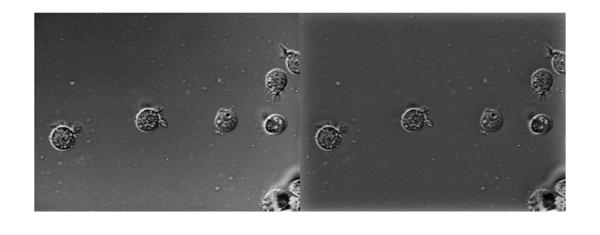Next time we will explore homomorphic filtering some more. We will see a slightly modified form of homomorphic filtering and also discuss why we might want to do filtering in the spatial domain.

Published with MATLAB® R2013a

|